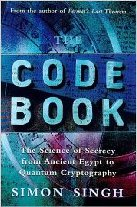# Four-Square Cipher

## Introduction §

The Four-square cipher encrypts pairs of letters (like playfair), which makes it significantly stronger than substitution ciphers etc. since frequency analysis becomes much more difficult.

Felix Delastelle (1840 - 1902) invented the four-square cipher, first published in a book in 1902. Delastelle was most famous for his invention of several systems of polygraphic substitution ciphers including bifid, trifid, and the four-square cipher.

For a guide on how to break the foursquare cipher using Simulated Annealing, see Cryptanalysis of the Foursquare Cipher.

## The Algorithm §

The four-square cipher uses four 5 by 5 matrices arranged in a square. Each of the 5 by 5 matrices contains 25 letters, usually the letter 'j' is merged with 'i' (wikipedia says 'q' is omitted, it is not very important since both q and j are rather rare letters). In general, the upper-left and lower-right matrices are the "plaintext squares" and each contain a standard alphabet. The upper-right and lower-left squares are the "ciphertext squares" and contain a mixed alphabetic sequence.

The ciphertext squares can be generated using a keyword (dropping duplicate letters), then fill the remaining spaces with the remaining letters of the alphabet in order. Alternatively the ciphertext squares can be generated completely randomly. The four-square algorithm allows for two separate keys, one for each of the two ciphertext matrices.

### Steps §

1. Break up the plaintext into bigrams i.e. ATTACK AT DAWN --> AT TA CK AT DA WN
An 'X' (or some other character) may have to be appended to ensure the plaintext is an even length.

2. Using the four 'squares', two plain alphabet squares and two cipher alphabet squares, locate the bigram to encrypt in the plain alphabet squares. The example below enciphers the bigram 'AT'. The first letter is located from the top left square, the second letter is located in the bottom right square.

```
a b c d e   Z G P T F
f g h i k   O I H M U
l m n o p   W D R C N
q r s t u   Y K E Q A
v w x y z   X V S B L

M F N B D   a b c d e
C R H S A   f g h i k
X Y O G V   l m n o p
I T U E W   q r s t u
L Q Z K P   v w x y z
```
3. Locate the characters in the ciphertext at the corners of the rectangle that the letters 'AT' make:

```
a b c d e   Z G P T F
f g h i k   O I H M U
l m n o p   W D R C N
q r s t u   Y K E Q A
v w x y z   X V S B L

M F N B D   a b c d e
C R H S A   f g h i k
X Y O G V   l m n o p
I T U E W   q r s t u
L Q Z K P   v w x y z
```

4. Using the above keys, the bigram 'AT' is encrypted to 'TI'.

The text 'attack at dawn', with the keys 'zgptfoihmuwdrcnykeqaxvsbl' and 'mfnbdcrhsaxyogvituewlqzkp', becomes:

```ATTACKATDAWN
TIYBFHTIZBSY
```

## JavaScript Example of the Foursquare Cipher §

This is an implementation of the Foursquare cipher in javascript. The keysquares are written out as a 25 character string, instead of a 5 by 5 array. Plaintext

keysquare 1 =
keysquare 2 =

Ciphertext

## Other Implementations §

To encipher your own messages in python, you can use the pycipher module. To install it, use pip install pycipher. To encipher messages with the Foursquare cipher (or another cipher, see here for documentation):

```>>>from pycipher import Foursquare
>>>fs = Foursquare('zgptfoihmuwdrcnykeqaxvsbl','mfnbdcrhsaxyogvituewlqzkp')
>>>fs.encipher('defend the east wall of the castle')
'FBUMCNESFDPIKKZXCXMIUNZNQUNM'
>>>fs.decipher('FBUMCNESFDPIKKZXCXMIUNZNQUNM')
'DEFENDTHEEASTWALLOFTHECASTLE'
```

## Cryptanalysis §

For a guide on how to break the foursquare cipher using Simulated Annealing, see Cryptanalysis of the Foursquare Cipher.

The four-square cipher can be easily cracked with enough ciphertext. It is quite simple to determine the key if both plaintext and ciphertext are known, and for this reason guessing parts of the plaintext is a very effective way of cracking this cipher. If a portion of the plaintext is known or can be guessed this should be exploited first to determine as much of the key as possible, then more guessing can be applied or other techniques described below.

Compared to the Playfair cipher, a four-square cipher will not show reversed ciphertext digraphs for reversed plaintext digraphs (e.g. the digraphs AB BA would encrypt to some pattern XY YX in Playfair, but not in four-square). This, of course, is only true if the two keywords are different. Another difference between four-square and Playfair which makes four-square a stronger encryption is the fact that double letter digraphs will occur in four-square ciphertext. 

The four-square cipher is a stronger cipher than Playfair, but it is more cumbersome because of its use of two keys and preparing the encryption/decryption sheet can be time consuming. Given that the increase in encryption strength afforded by four-square over Playfair is marginal and that both schemes are easily defeated if sufficient ciphertext is available, Playfair was much more common.

A good tutorial on reconstructing the key for a four-square cipher can be found in chapter 7, "Solution to Polygraphic Substitution Systems," of Field Manual 34-40-2, produced by the United States Army. Another source is provided in lecture 17 of the LANIKI Crypto Course Lessons Lecture 17.

## ContentsThe Code Book: The Science of Secrecy from Ancient Egypt to Quantum Cryptography ASIN/ISBN: 978-1857028799 Simon Singh's 'The Code Book' is an excellent introduction to ciphers and codes Buy from Amazon.com